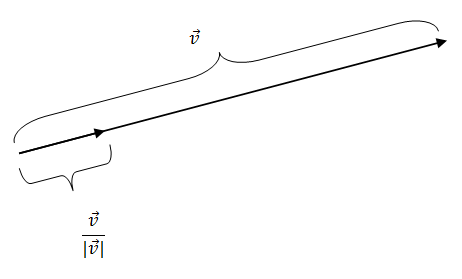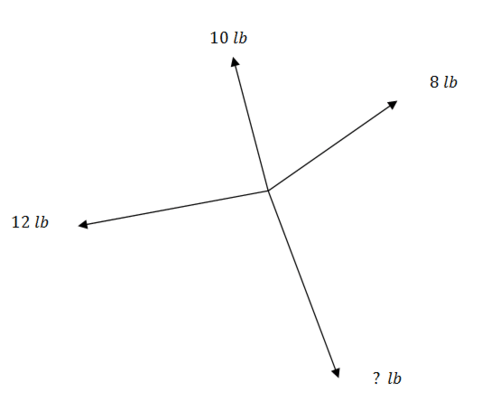7.3: Resolution of Vectors into Components

•• Contributed by CK12
• CK12

Sometimes working with horizontal and vertical components of a vector can be significantly easier than working with just an angle and a magnitude. This is especially true when combining several forces together.

Consider four siblings fighting over a candy in a four way tug of war. Lanie pulls with 8 Ib of force at an angle of $$41^{\circ}$$. Connie pulls with 10 Ib of force at an angle of $$100^{\circ}$$. Cynthia pulls with 12 Ib of force at an angle of $$200^{\circ}$$. How much force and in what direction does poor little Terry have to pull the candy so it doesn't move?

The Unit Vector and Component Form

A unit vector is a vector of length one. Sometimes you might wish to scale a vector you already have so that it has a length of one. If the length was five, you would scale the vector by a factor of $$\frac{1}{5}$$ so that the resulting vector has magnitude of $$1 .$$ Another way of saying this is that a unit vector in the direction of vector $$\vec{v}$$ is $$\frac{\vec{v}}{|\vec{v}|}$$There are two standard unit vectors that make up all other vectors in the coordinate plane. They are $$\vec{i}$$ which is the vector $$<1,0>$$ and $$\vec{j}$$ which is the vector $$<0,1>$$. These two unit vectors are perpendicular to each other. A linear combination of $$\vec{i}$$ and $$\vec{j}$$ will allow you to uniquely describe any other vector in the coordinate plane in component form. For instance the vector $$<5,3>$$ is the same as $$5 \vec{i}+3 \vec{j}$$.

Often vectors are initially described as an angle and a magnitude rather than in component form. Working with vectors written as an angle and magnitude requires extremely precise geometric reasoning and excellent pictures. One advantage of rewriting the vectors in component form is that much of this work is simplified. Remember that component form is the form $$<x, y>$$ and to translate from magnitude $$r$$ and direction $$\theta$$ to component form, use the relationship $$<r \cdot \cos \theta, r \cdot \sin \theta>=<x, y>$$. In this situation $$r$$ is the magnitude and $$\theta$$ is the direction.

Take a plane that has a bearing of $$60^{\circ}$$ and is going $$350 \mathrm{mph} .$$ To find the component form of the velocity of the airplane, note that a bearing of $$60^{\circ}$$ is the same as a $$30^{\circ}$$ on the unit circle. This corresponds to the point $$\left(\frac{\sqrt{3}}{2}, \frac{1}{2}\right)$$ which is the same as $$(\cos 30, \sin 30)$$. When written as a vector $$<\frac{\sqrt{3}}{2}, \frac{1}{2}>$$ is a unit vector
because it has magnitude 1. Now you just need to scale by a factor of 350 and you get your answer of $$<175 \sqrt{3}, 175>$$. Thus, the velocity of the airplane in component form is $$<175 \sqrt{3}, 175>$$. This is the same as using the relationship $$<r \cdot \cos \theta, r \cdot \sin \theta>=<x, y>$$.

Examples

Example 1

Earlier, you were asked to consider four siblings fighting over a candy in a four way tug of war. Lanie pulls with 8 Ib of force at an angle of $$41^{\circ}$$. Connie pulls with 10 Ib of force at an angle of $$100^{\circ}$$. Cynthia pulls with 12 Ib of force at an angle of $$200^{\circ}$$. How much force and in what direction does poor little Terry have to pull the candy so it doesn't move?To add the three vectors together would require several iterations of the Law of Cosines.  Instead, write each vector in component form and set equal to a zero vector indicating that the candy does not move.

$$\vec{L}+\overrightarrow{C O N}+\overrightarrow{C Y N}+\vec{T}=<0,0>$$

\begin{aligned} &<8 \cdot \cos 41^{\circ}, 8 \cdot \sin 41^{\circ}>+<10 \cdot \cos 100^{\circ}, 10 \cdot \sin 100^{\circ}>\\+&<12 \cdot \cos 200^{\circ}, 12 \cdot \sin 200^{\circ}>+\vec{T}=<0,0>\end{aligned}

Use a calculator to add all the $$x$$ components and bring them to the far side and the $$y$$ components and then subtract from the far side to get:

$$\vec{T} \approx<6.98,-10.99>$$

Turning this component vector into an angle and magnitude yields how hard and in what direction he would have to pull. Terry will have to pull with about 13 Ib of force at an angle of $$302.4^{\circ}$$.

Example 2

Consider the plane that has a bearing of $$60^{\circ}$$ and is going $$350 \mathrm{mph}$$. If there is wind blowing with the bearing of $$300^{\circ}$$ at $$45 \mathrm{mph},$$ what is the component form of the total velocity of the airplane?

A bearing of $$300^{\circ}$$ is the same as $$150^{\circ}$$ on the unit circle which corresponds to the point $$\left(-\frac{\sqrt{3}}{2}, \frac{1}{2}\right)$$. You can now write and then scale the wind vector.

$$45 \cdot<-\frac{\sqrt{3}}{2}, \frac{1}{2}>=<-\frac{45 \sqrt{3}}{2}, \frac{45}{2}>$$

Since both the wind vector and the velocity vector of the airplane are written in component form, you can simply sum them to find the component vector of the total velocity of the airplane.

$$<175 \sqrt{3}, 175>+<-\frac{45 \sqrt{3}}{2}, \frac{45}{2}>=<\frac{305 \sqrt{3}}{2}, \frac{395}{2}>$$

Example 3

Consider the plane from Example 2 with the same wind and velocity. Find the actual ground speed and direction of the plane (as a bearing).

Since you already know the component vector of the total velocity of the airplane, you should remember that these components represent an $$x$$ distance and a $$y$$ distance and the question asks for the hypotenuse.

\begin{aligned}\left(\frac{305 \sqrt{3}}{2}\right)^{2}+\left(\frac{395}{2}\right)^{2} &=c^{2} \\ 329.8 & \approx c \end{aligned}

The airplane is traveling at about 329.8 mph.

Since you know the $$x$$ and $$y$$ components, you can use tangent to find the angle. Then convert this angle into bearing.

\begin{aligned} \tan \theta &=\frac{\left(\frac{395}{2}\right)}{\left(\frac{305 \sqrt{3}}{2}\right)} \\ \theta & \approx 36.8^{\circ} \end{aligned}

An angle of $$36.8^{\circ}$$ on the unit circle is equivalent to a bearing of $$53.2^{\circ}$$.

Note that you can do the entire problem in bearing by just switching sine and cosine, but it is best to truly understand what you are doing every step of the way and this will probably involve always going back to the unit circle.

For Examples 4 and 5, use the following information:

$$\vec{v}=<2,-5>, \vec{u}=<-3,2>, \vec{t}=<-4,-3>, \vec{r}=<5, y>$$
$$B=(4,-5), P=(-3,8)$$

Example 4

Find the unit vectors in the same direction as $$\vec{u}$$ and $$\vec{t}$$.

To find a unit vector, divide each vector by its magnitude.

$$\frac{\vec{u}}{|\vec{u}|}=<\frac{-3}{\sqrt{13}}, \frac{2}{\sqrt{13}}>, \frac{\vec{t}}{|\vec{t}|}=<\frac{-4}{5}, \frac{-3}{5}>$$

Example 5

Find the point 10 units away from $$B$$ in the direction of $$P$$.

The vector $$\overrightarrow{B P}$$ is $$<-7,13>$$. First take the unit vector and then scale it so it has a magnitude of $$10 .$$

\begin{aligned} \frac{B P}{|B P|} &=<\frac{-7}{\sqrt{218}}, \frac{13}{\sqrt{218}}>\\ 10 \cdot \frac{B P}{|B P|} &=<\frac{-70}{\sqrt{218}}, \frac{130}{\sqrt{218}}>\end{aligned}

You end up with a vector that is ten units long in the right direction. The question asked for a point from $$B$$ which
means you need to add this vector to point $$B$$.

$$(4,-5)+<\frac{-70}{\sqrt{218}}, \frac{130}{\sqrt{218}}>\approx(-0.74,3.8)$$

Review

Use the following defined vectors and points to answer $$1-8$$.

$$\vec{v}=<1,-3>, \vec{u}=<2,5>, \vec{t}=<9,-1>, \vec{r}=<2, y>$$

$$A=(-3,2), B=(5,-2)$$

1. Solve for $$y$$ in vector $$\vec{r}$$ to make $$\vec{r}$$ perpendicular to $$\vec{t}$$.

2. Find the unit vector in the some direction os $$\vec{u}$$.

3. Find the unit vector in the some direction as $$\vec{t}$$.

4. Find the unit vector in the some direction os $$\vec{v}$$

5. Find the unit vector in the some direction os $$\vec{r}$$.

6. Find the point exactly 3 units owey from $$A$$ in the direction of $$B$$.

7. Find the point exoctly 6 units owry from $$B$$ in the direction of $$A$$.

8. Find the point exoctly 5 units owoy from $$A$$ in the direction of $$B$$.

9. Jock ond Jill went up a hill to fetch o poil of woter. When they got to the top of the hill, they were very thirsty so they each pulled on the bucket. Jill pulled ot $$30^{\circ}$$ with 20 lbs of force. Jock pulled ot $$45^{\circ}$$ with 28 lbs of force. What is the resulting vector for the bucket?

10. A plane is flying on a bearing of $$60^{\circ}$$ at $$400 \mathrm{mph}$$. Find the component form of the velocity of the plane. What does the component form tell you?
11. A baseball is thrown at a $$70^{\circ}$$ angle with the horizontal with an initial speed of 30 mph. Find the component form of the initial velocity.
12. A plane is flying on a bearing of $$200^{\circ}$$ at $$450 \mathrm{mph}$$. Find the component form of the velocity of the plane.

13. A plane is flying on a bearing of $$260^{\circ}$$ at 430 mph. At the same time, there is a wind blowing at a bearing of $$30^{\circ}$$ at $$60 \mathrm{mph} .$$ What is the component form of the velocity of the plane?

14. Use the information from the previous problem to find the actual ground speed and direction of the plane.

15. Wind is blowing at a magnitude of 40 mph with an angle of $$25^{\circ}$$ with respect to the east. What is the velocity of the wind blowing to the north? What is the velocity of the wind blowing to the east?